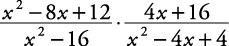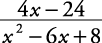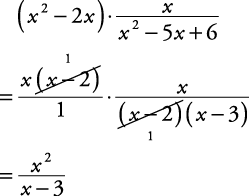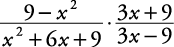## Multiplying Rational Expressions

To multiply rational expressions:

1. Completely factor all numerators and denominators.

2. Reduce all common factors.

3. Either multiply the denominators and numerators or leave the answer in factored form.

##### Example 1

Simplify.This last answer could be either left in its factored form or multiplied out. If multiplied out, it becomes##### Example 2

Simplify.##### Example 3

Simplify.